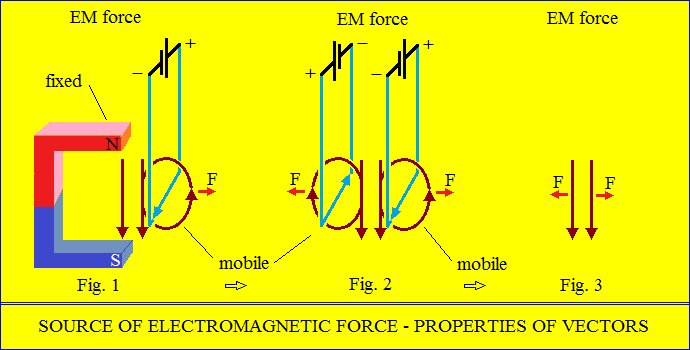Electromagnetism
Certainly, the magnetic field of the earth exists.
The magnetic field of the earth can only be produced by electricity.
These phenomena are found in all cosmic bodies.
The question is how electromagnetism is produced.
What are the electromagnetic forces?
Electromagnetic force is the interaction between the field of a permanent magnet
and the field of an electric current.
More specifically, between two magnetic fields.
And fundamentally, between two parallel magnetic vectors.
It follows that EM forces are interactions between vector properties.
First, we need to understand how to interpret electromagnetic interaction.
Fig. 1 - the fixed magnet field, exerts a force that moves a mobile frame with "electric current".
Fig. 2 - two with "electric current" mobile frames are rejecting each other.
It is the third principle of Newton's mechanics.
Fig. 3 - the two frames are reduced to two parallel vectors, in the same direction and sense.
So, the electromagnetic forces are derived from the properties of the vectors,
as the Third Principle of Newton's Mechanics says.<
>